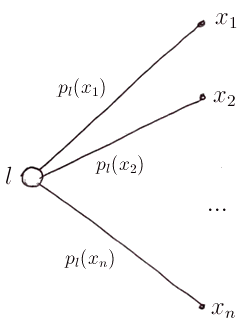# ML Wiki

## Decision Tree

This is a tool for modeling decision taking process for Decision Under Risk

## Lotteries

Notation:

• $A$ - set of alternatives
• $X = \{x_1, ..., x_n)\}$ - a finite set of consequences
• could be $X \subseteq \mathbb{R}$ - e.g. money, etc

### Simple Lotteries

A simple lottery $l$ on $X$ is

• a discrete Random Value on $X$
• $l = \{(x_1, p_1), (x_2, p_2), ..., (x_n, p_n) \}$
• $x_i$ is a consequence, $p_i$ is the probability that $x_i$ will happen
• this is a simple model: it depends only on one set of consequences

Visual representation:

•### Set of Lotteries

But we can also have a lottery over lotteries

A set of lotteries:

• simple lotteries on $X$
• first-order lotteries on simple lotteries
• second-order lotteries on first-order lotteries
• etc

Notation

• Let $L(X)$ denote the set of all lotteries at all finite orders
• $L(X)$ includes all lotteries that correspond to implementation of alternatives from $A$
• $l \in L(X)$ a lottery from $L(X)$ - can be simple or not
• $p_l(x)$ is the probability to face consequence $x$ in lottery $l$

## Decision Trees

Decision Trees have three kinds of nodes:

• decision nodes
• here the decision maker has to choose which action to implement
• chance nodes
• at a chance node the Nature chooses a branch according to the probability distribution
• this is a lottery of higher order
• terminal nodes
• single lotteries out of $L(X)$

## Comparing Lotteries

To be able to decide on the decision nodes, a decision maker needs to be able to compare different lotteries